# Definition and Properties of Trees

Tree is a discrete structure that represents hierarchical relationships between individual elements or nodes. A tree in which a parent has no more than two children is called a binary tree.

## Tree and its Properties

Definition − A Tree is a connected acyclic undirected graph. There is a unique path between every pair of vertices in G. A tree with N number of vertices contains (N-1) number of edges. The vertex which is of 0 degree is called root of the tree. The vertex which is of 1 degree is called leaf node of the tree and the degree of an internal node is at least 2.

Example − The following is an example of a tree −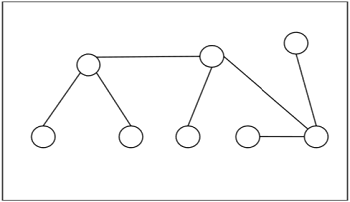## Centers and Bi-Centers of a Tree

The center of a tree is a vertex with minimal eccentricity. The eccentricity of a vertex X in a tree G is the maximum distance between the vertex X and any other vertex of the tree. The maximum eccentricity is the tree diameter. If a tree has only one center, it is called Central Tree and if a tree has only more than one centers, it is called Bi-central Tree. Every tree is either central or bi-central.

## Labeled Trees

Definition − A labeled tree is a tree the vertices of which are assigned unique numbers from 1 to n. We can count such trees for small values of n by hand so as to conjecture a general formula. The number of labeled trees of n number of vertices is nn-2. Two labeled trees are isomorphic if their graphs are isomorphic and the corresponding points of the two trees have the same labels.

## Example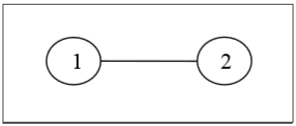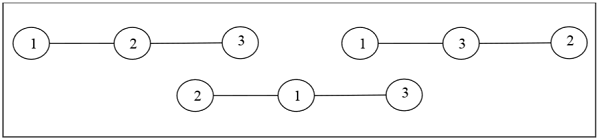## Unlabeled Trees

Definition − An unlabeled tree is a tree the vertices of which are not assigned any numbers. The number of labeled trees of n number of vertices is $\frac {(2n)!}{ (n+1)!n! }$ (nth Catalan number)

## Example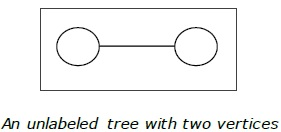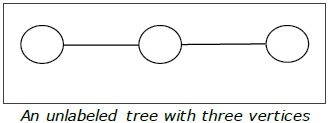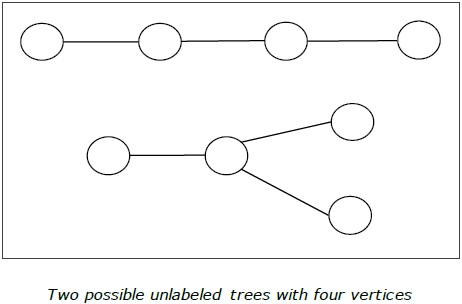## Rooted Tree

A rooted tree G is a connected acyclic graph with a special node that is called the root of the tree and every edge directly or indirectly originates from the root. An ordered rooted tree is a rooted tree where the children of each internal vertex are ordered. If every internal vertex of a rooted tree has not more than m children, it is called an m-ary tree. If every internal vertex of a rooted tree has exactly m children, it is called a full m-ary tree. If m = 2, the rooted tree is called a binary tree.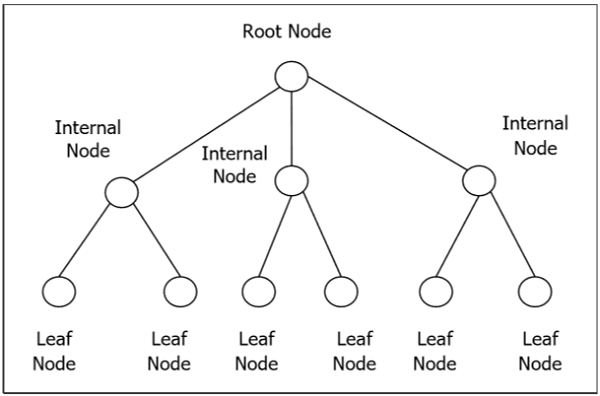## Binary Search Tree

Binary Search tree is a binary tree which satisfies the following property −

• X in left sub-tree of vertex V, Value(X) ≤ Value (V)
• Y in right sub-tree of vertex V, Value(Y) ≥ Value (V)

So, the value of all the vertices of the left sub-tree of an internal node V are less than or equal to V and the value of all the vertices of the right sub-tree of the internal node V are greater than or equal to V. The number of links from the root node to the deepest node is the height of the Binary Search Tree.

## Example### Algorithm to search for a key in BST

BST_Search(x, k)
if ( x = NIL or k = Value[x] )
return x;
if ( k < Value[x])
return BST_Search (left[x], k);
else
return BST_Search (right[x], k)

### Complexity of Binary search tree

Average CaseWorst case
Space ComplexityO(n)O(n)
Search ComplexityO(log n)O(n)
Insertion ComplexityO(log n)O(n)
Deletion ComplexityO(log n)O(n)